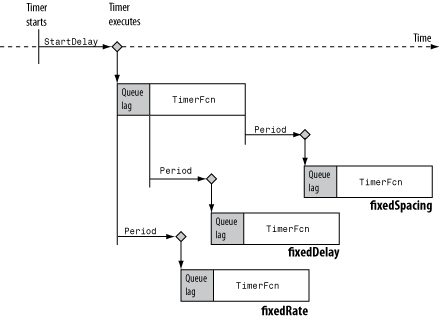# set

## 语法

``set(t) ``
``propStruct = set(t) ``
``set(t,Name) ``
``propCell = set(t,Name) ``
``set(t,Name,Value) ``
``set(t,S) ``
``set(t,PN,PV) ``

## 说明

``set(t) ` 显示计时器对象 `t` 的所有可配置属性的属性名称及其可能值。 `

``propStruct = set(t) ` 在 `struct` 中返回这些值。 `

``set(t,Name) ` 显示计时器对象 `t` 的指定属性 `Name` 的可能值。 `

``propCell = set(t,Name) ` 在元胞中返回这些值。`

``set(t,Name,Value) ` 设置由一个或多个 `Name,Value` 对组参数指定的属性。`t` 可以是单个计时器对象或计时器对象向量，对于后者，`set` 会为所有计时器对象 `t` 配置属性值。`

``set(t,S) ` 使用 `S` 中指定的值配置 `t` 的属性，其中 `S` 是一个字段名称为对象属性名称的结构体。`

``set(t,PN,PV) ` 针对计时器对象 `t` 将字符向量元胞数组 `PN` 中指定的属性配置为元胞数组 `PV` 中的对应值。 `

## 输入参数

 `t` 类 `timer` 的对象。 `S` 结构体，其字段名称等于 `timer` 属性名称。 `PN,PV` 字符向量元胞数组 `PN` 及对应的值元胞数组 `PV`。`PN` 元胞数组必须是一个 1×N 或 N×1 数组。如果 `t` 是一个计时器对象数组，则 `PV` 可以是一个 M×N 元胞数组，其中 M 等于 `t` 的长度，N 等于 `PN` 的长度。在这种情况下，每个计时器对象都会用一组不同值更新 `PN` 中包含的属性名称列表。

### 名称-值对组参数

`BusyMode`

`BusyMode`

`'drop'`

`'error'`

`'queue'`

`ErrorFcn`

• 如果您使用字符向量或字符串标量指定此属性，则当 MATLAB® 执行回调时，它会对字符向量中包含的 MATLAB 代码求值。

• 如果您使用函数句柄指定此属性，当 MATLAB 执行回调时，它会将 `timer` 对象和事件结构体传递给回调函数。事件结构体的 `Type` 字段中包含事件的类型，`Data` 字段中包含事件的时间。

• 如果回调函数接受除 `timer` 对象和事件数据以外的参数，请将此属性指定为包含函数句柄和其他参数的元胞数组。

`ExecutionMode`

`'singleShot'`

`'fixedRate'`

`'fixedDelay'`

`'fixedSpacing'`

• `'singleShot'``timer` 类的单次执行模式，并且是默认值。• `'fixedDelay'``'fixedRate'``'fixedSpacing'` 是三个受支持的多次执行模式。这些模式定义 `Period` 属性的起点。`Period` 属性指定执行之间的间隔时间（保持不变）。只有执行起点是不同的。`Name`

`ObjectVisibility`

`Period`

`StartDelay`

`StartFcn`

• 如果您使用字符向量或字符串标量指定此属性，则当 MATLAB 执行回调时，它会对字符向量中包含的 MATLAB 代码求值。

• 如果您使用函数句柄指定此属性，当 MATLAB 执行回调时，它会将 `timer` 对象和事件结构体传递给回调函数。事件结构体的 `Type` 字段中包含事件的类型，`Data` 字段中包含事件的时间。

• 如果回调函数接受除 `timer` 对象和事件数据以外的参数，请将此属性指定为包含函数句柄和其他参数的元胞数组。

`StopFcn`

• 如果您使用字符向量或字符串标量指定此属性，则当 MATLAB 执行回调时，它会对字符向量中包含的 MATLAB 代码求值。

• 如果您使用函数句柄指定此属性，当 MATLAB 执行回调时，它会将 `timer` 对象和事件结构体传递给回调函数。事件结构体的 `Type` 字段中包含事件的类型，`Data` 字段中包含事件的时间。

• 如果回调函数接受除 `timer` 对象和事件数据以外的参数，请将此属性指定为包含函数句柄和其他参数的元胞数组。

• 调用计时器 `stop` 方法。

• 计时器完成执行 `TimerFcn`。换句话说，`TasksExecuted` 的值达到 `TasksToExecute` 设置的限值。

• 出现错误。首先调用 `ErrorFcn` 回调，然后调用 `StopFcn` 回调。

`Tag`

`TasksToExecute`

`TimerFcn`

• 如果您使用字符向量或字符串标量指定此属性，则当 MATLAB 执行回调时，它会对字符向量中包含的 MATLAB 代码求值。

• 如果您使用函数句柄指定此属性，当 MATLAB 执行回调时，它会将 `timer` 对象和事件结构体传递给回调函数。事件结构体的 `Type` 字段中包含事件的类型，`Data` 字段中包含事件的时间。

• 如果回调函数接受除 `timer` 对象和事件数据以外的参数，请将此属性指定为包含函数句柄和其他参数的元胞数组。

`UserData`

## 输出参数

 `propStruct` `t` 的可配置属性，以结构体形式返回。`propStruct` 的字段名称为 `t` 的属性名称，`propStruct` 的关联值为可能的属性值元胞数组。如果 `t` 中的属性没有一组有限的可能值，则 `propStruct` 中的属性值为空元胞数组。 `propCell` 给定属性名称的可能值，以字符向量元胞数组形式返回。如果该属性没有一组有限的可能值，`set` 将返回空元胞数组。

## 示例

```t = timer; set(t)```
``` Name: {} Tag: {} ObjectVisibility: {2x1 cell} TasksToExecute: {} StartFcn: {} StopFcn: {} ErrorFcn: {} TimerFcn: {} StartDelay: {} Period: {} BusyMode: {3x1 cell} ExecutionMode: {4x1 cell} UserData: {} ```

`out = set(t)`
```out = struct with fields: Name: {} Tag: {} ObjectVisibility: {2x1 cell} TasksToExecute: {} StartFcn: {} StopFcn: {} ErrorFcn: {} TimerFcn: {} StartDelay: {} Period: {} BusyMode: {3x1 cell} ExecutionMode: {4x1 cell} UserData: {} ```

`delete(t)`

```t = timer; set(t,'BusyMode')```
``` 3x1 cell array {'drop' } {'queue'} {'error'} ```

`set(t,'ErrorFcn')`
``` 0x0 empty cell array ```

`out1 = set(t,'BusyMode')`
```out1 = 3x1 cell {'drop' } {'queue'} {'error'} ```
`out2 = set(t,'ErrorFcn')`
```out2 = 0x0 empty cell array ```

`delete(t)`

```t = timer; set(t,'ObjectVisibility','off') get(t,'ObjectVisibility')```
```ans = 'off' ```
`delete(t)`

```s.BusyMode = 'queue'; s.ExecutionMode = 'fixedDelay'; s.ObjectVisibility = 'off'```
```s = struct with fields: BusyMode: 'queue' ExecutionMode: 'fixedDelay' ObjectVisibility: 'off' ```

```t = timer; get(t,{'BusyMode','ExecutionMode','ObjectVisibility'})```
```ans=1×3 cell array {[drop]} {[singleShot]} {'on'} ```
```set(t,s) get(t,{'BusyMode','ExecutionMode','ObjectVisibility'})```
```ans=1×3 cell array {[queue]} {[fixedDelay]} {'off'} ```
`delete(t)`

```nameArr = {'BusyMode','ExecutionMode','Period'}; valArr = {'queue','fixedDelay',3}; t = timer; get(t,nameArr)```
```ans=1×3 cell array {[drop]} {[singleShot]} {} ```

```set(t,nameArr,valArr) get(t,nameArr)```
```ans=1×3 cell array {[queue]} {[fixedDelay]} {} ```
`delete(t)`

```tArr = [timer timer timer]; nameArr = {'BusyMode','ExecutionMode','UserData'}; get(tArr,nameArr)```
```ans=3×3 cell array {[drop]} {[singleShot]} {0x0 double} {[drop]} {[singleShot]} {0x0 double} {[drop]} {[singleShot]} {0x0 double} ```

```valArr = {'queue','fixedDelay',3;... 'error','fixedSpacing',42;... 'drop','fixedRate','hello'};```

```set(tArr,nameArr,valArr) get(tArr,nameArr)```
```ans=3×3 cell array {[queue]} {[fixedDelay ]} {[ 3]} {[error]} {[fixedSpacing]} {[ 42]} {[drop ]} {[fixedRate ]} {'hello'} ```

`delete(tArr)`